Please wait, while our marmots are preparing the hot chocolate…
## {image-full top-left darkened /black-bg /no-status /first-slide title-slide fancy-slide bot}
- - - - 5 points {notes} - who - worked on unsup learning for 5-6 y - you'll get an overview of Graphical models vs NN, for unsupervised - you'll get a taste of interesting recent works - NIPS 2016 last week## Disclaimer {infobox image-full top-right darkened /black-bg /no-status}
- Some notations are atypical. // due to the mix of domains - I will, almost surely, skip sections. - Don't hesitate to ask questions lives. ## TITLE {#plan plan overview /with-ujm} - Unsupervised Representation Learning {intro} - Notations and problem formulation {setup} - Probabilistic (graphical) models {probmod} - Auto-encoders {autoenc} - Generative Adversarial Networks {gan} - adversarial examples and training - GANs - Focus on … {focuses} - optimization {focus optim} - space and time convolutions {focus conv} - *depth {focus depth}*, *breadth/width {focus width}* - semantics {focus semantics} - sequential/temporal aspects {focus temporal} - recent GAN[s](#recentgans) {focus recentgans} - Wrap up {conclusion} # @copy:#plan: %+class:highlight: .intro ## Representation Learning at Hubert Curien // supervised at Data Intelligence, mostly equivalent to learning @svg: repr/representation-di.svg 800 500 - @anim: #tasks |#data-low |#data-mid |#data-high |#methods-title |#methods-mining |#methods-metric |#methods-local |#methods-grammar |#methods-deep ## Unsupervised (Representation) Learning - No labels available - Learning intermediate features or representations - Task agnostic - Related to (data) density estimation - Related to compression ## Example: motif mining in videos / temporal data @svg: motif-mining/motif-mining-task.svg 750 400 - @anim: #layer1 + -#init | #layer2 | #layer3 | #layer7 | #layer4 | #layer6 | #layer5 - Key points: structure? compression? density estimation? {slide} // st {hard, related, that I know} well-enough - {notes} - n. relation to compression, ... - n. notion of need to have a some structure/assumptions/priors - n. structured data probability density estimation - n. something that is {hard, related, that I know} well-enough # @copy:#plan: %+class:highlight: .setup # Notations and problem formulation {#setup} ## Notations and Problem Formulation - Notations - $x$ : data (observations) - $y$ : value to predict (for supervised cases) - $z$ : unknown, unobserved latent information - $\theta$ (or $W$) : model parameters // will come back on the differences z vs θ - Unsupervised learning - only $x$ is given - need to find the parameters ($\theta$, $W$) - may want to further infer the latent variables ($z$) # @copy:#plan: %+class:highlight: .probmod # Probabilistic (graphical) models {#probmod} ## Generative Model, Parameters, Latent Vars… - Observations / Data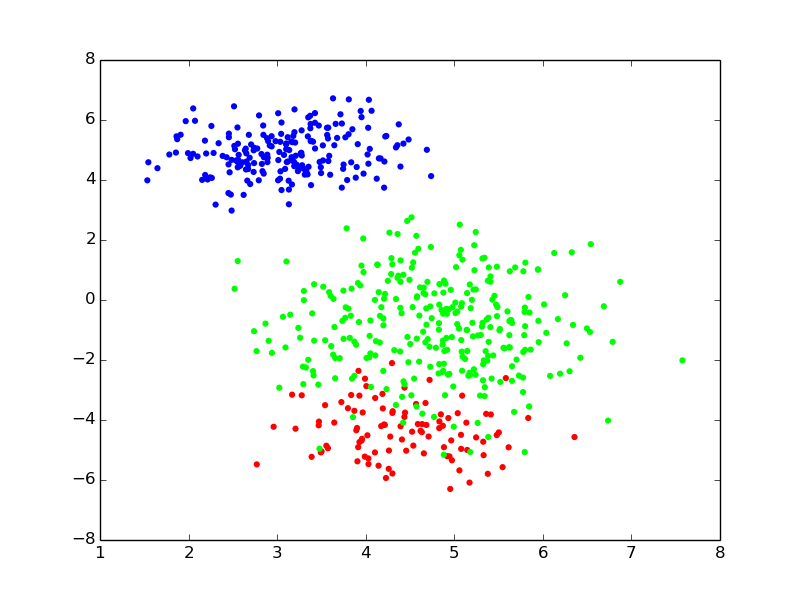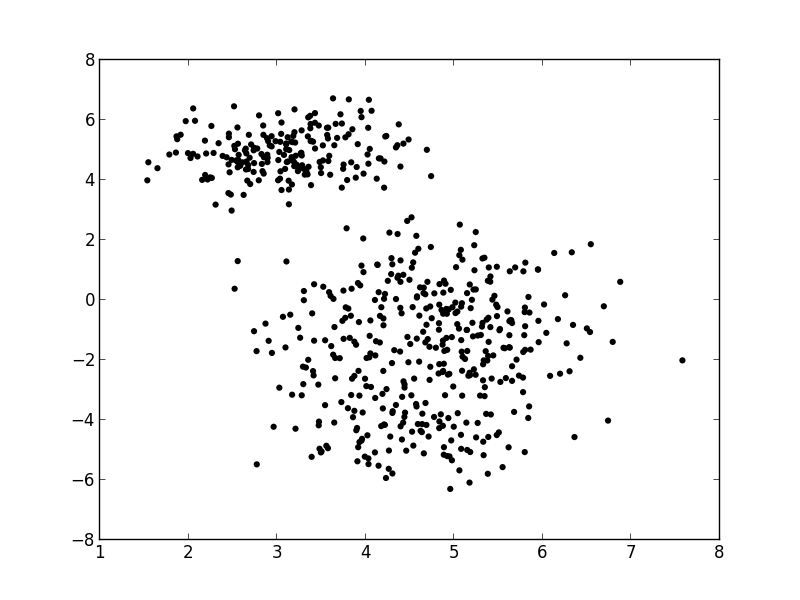- Supposition, we have a mixture of 3 gaussians {slide} - Challenge {slide} - gaussians have unknown *parameters* - which point belongs to which component is *not observable* - @anim: .first ## Probabilistic Modeling: principle {libyli} - Adopting a generative approach - think about how the world generated the data - describe it in a “generative model” - Formalize your assumptions about the observations (data) - choose/design a model - a model formulates how *some unknown variables* that are “responsible” for the *observations* (data) - set some priors on the unknown variables - Naming convention: different types of unknowns - parameters: unknown global parameters of the model - latent variables: unknown observation-specific variables // usually unknown - With a mixture of Gaussians - parameters: mean and covariances (and weight) of all Gaussians - latent variables: which Gaussian each data points comes from ## Probabilistic Model Learning {libyli} - The model is generative - describes how the data ($x$) gets generated - “forward model” - the probability of the observations: $p(x | \theta)$ - Finding the unknowns (parameters, latent var.) is challenging - reversing the generative process - finding (or maximizing) $p(\theta | x)$ or $p(\theta, z | x)$ or $p(z | x, \theta)$ - high dimensional parameter/latent spaces - highly non-convex functions ## M1 − PCA: intuition @svg: media/wikipedia-pca.svg 800 500 - @anim: #patch_3 | #patch_4 ## M1: PCA @svg: media/wikipedia-pca.svg 200 200 {model} @svg: graphs/theta-x.svg 100 250 {model m11 clearright} @svg: graphs/x-f-theta.svg 100 250 {model minv} // @svg: media/factor.svg 40 300 {model m12} - Principle Component Analysis (eigen-*) - dimensionality reduction - capture the maximum amount of data variance - PCA probabilistic view {libyli} - observations come from a single low-dimensional gaussian distribution - ... and are transformed with a linear transformation (rotation + scale), - ... and have added noise noisy - @anim: .m11 - Over-generic graphical representation {slide} - $\theta$ is linear transformation - data points $x$ depend on $\theta$ - no *explicit* latent variables *{ico-pencil}* - @anim: .minv - Inference problem: $f$ {slide} - dedicated algorithms
(covariance matrix eigenvalues, iterative methods, …) ## M2 − Topic Modeling: matrix factorization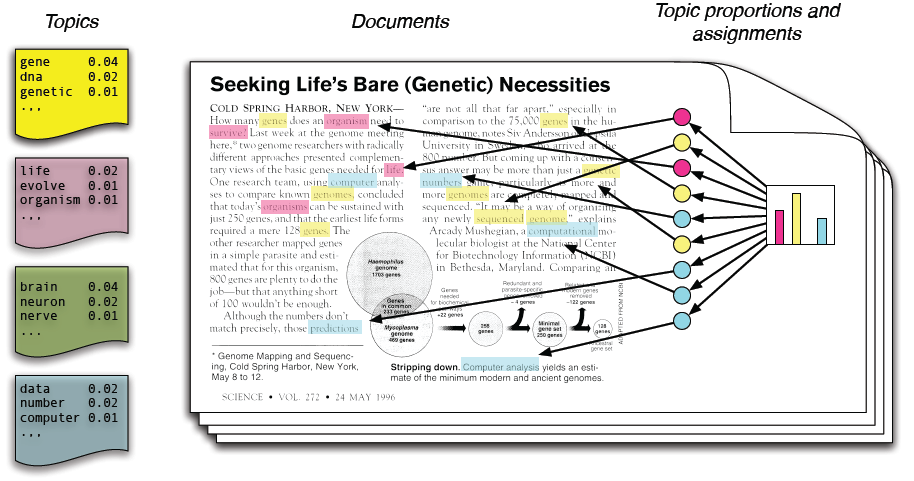- Probabilistic Latent Semantic Analysis (PLSA) - matrix decomposition {step2} - non-negative {step2} - probabilistic formulation {step2} - $p(w|d) = \sum_z p(w|z) \times p(z|d)$ {step2} - or $x^i = \theta^T \cdot z^i$ (for a document $i$) {step3} - @anim: .svg1 | #documents | #topics - @anim: .step2 | .step3 @svg: media/matrix-decomposition.svg 700 200 {svg1} ## M2: Topic Models {libyli} // our notation in this pres is highly confusion with standards of this domain @svg: graphs/theta-x.svg 50 200 {model m21} @svg: graphs/thetaz-x.svg 130 200 {model m22 clearright} @svg: graphs/x-f-thetaz.svg 130 200 {model m23} - LDA, topic models […](file:///home/twilight/doc/PublicationsAndPresentations/2012-cpms/day-11/cpms-lecture-11-topic-models.html#slide-4) - Latent Dirichlet Allocation - mixture of discrete distributions (categorical/multinomial) - Bayesian formulation of // won't go into details about bayesian - LSA, LSI (Latent Semantic Indexing) // we don't distinguish pLSA/LDA here - Probabilistic formulation of - NMF (non-negative matrix factorization) - @anim: .m21 - $x^i = \theta^T \cdot z^i$ (for a document $i$) *{ico-pencil}* - @anim: .m22 | .m23 - Learning/Inference, $f$ - Gibbs sampling - EM: expectation maximization - variational inference # @copy:#plan: %+class:highlight: .autoenc # Auto-encoders (not yet) {autoenc} ## Feed-forward Neural Networks (supervised) {libyli} @svg: graphs/ffnet.svg 120 400 {model} - Supervised learning (regression, classification, …) - the $x$ are given - the corresponding labels $y$ are given - Building blocks of “neural nets” - a neuron computes a weighted sum of its inputs - the sum is followed by an “activation” $\sigma$ - weights are learned ($W$) - $f^o(x^i, W) = \sigma\left( \sum_d W_{o,d} \times x^i_d \right) = \sigma\left( W_{o,.} ^T \cdot x^i\right)$ - Define a network architecture (class of functions) - number and dimension of layers - activation functions (sigmoid, tanh, ReLU, …) - … actually any composition of differentiable functions - Learning with stochastic gradient descent (SGD) and variants ## M3: Autoencoders {libyli} @svg: graphs/autoenc.svg 120 500 {model m31} - Idea: use a feed-forward approach - … for unsupervised learning (no labels) - to learn a compact data representation - Principle *{ico-pencil}* - try to predict the input form the input - have a latent **bottleneck**: limited model capacity - **encoder** $f$: from the input $x$ to the latent $z$ - **decoder** $g$: from the latent $z$ to the input $x$ - @anim: .m31, .cup - Learning principles of $f$ and $g$ - mean square reconstruction error: $\left\| g(f(x)) - x \right\|^2$ - SGD (like any neural net) - sparsifying regularization: sparse activations ($z$, $f(x)$) - add noise to the input (denoizing autoencoders)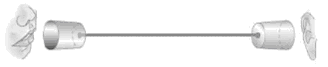n. RBM ? {notes} n. VAE ? {notes} # @copy:#plan: %+class:highlight: .gan # Generative Adversarial Networks {#gan} ## Adversarial Examples (Goodfellow, 2014) {libyli} // ostrich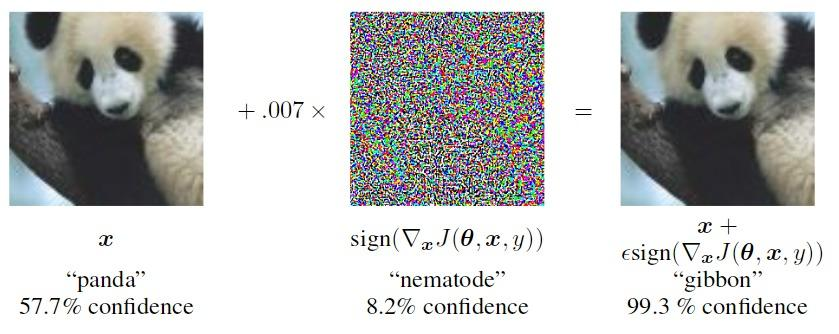- @anim: %attr:.hasFS:height:200 - In high dimensional spaces - a huge part of the input space is never seen / irrelevant - models are easy to fool - models are wrongly calibrated (bad confidence estimation) - Goal - build machine learning methods robust to adversarial examples - (relation to anomaly detection) - Idea of adversarial training - generate adversarial examples automatically - train also using these examples ## GAN Intuition {infobox image-full top-left darkened /black-bg /no-status}
- Ongoing struggle between two players: - one that makes fake samples, - one that tries to detect them. ## M4: Generative Adversarial Networks {libyli} @svg: graphs/ganright.svg 90 500 {model heightauto} @svg: graphs/ganleft.svg 90 482 {model heightauto alignbottom} - Principle: train two networks - $G$: to generate samples from noise - $D$: to discriminate between true and generated samples - NB: $G$ will try to fool $D$ - Elements *{ico-pencil}* - $x$: a training sample (real) - $z$: a random point in a latent space - $\tilde{x}{}$: a generated sample (fake) - $y$: a binary “fake” ($0$) or “real” ($1$) value - GAN is a minimax game - $\min_G \max_D V(D, G)$ - $V(D, G) = \; \mathbb{E}_{x} [log( D(x) ) ] + \mathbb{E}_z [log(1 - D(G(z))) ]$ ## GAN Target {libyli} @svg: graphs/ganright.svg 90 500 {model heightauto} - GAN optimization is a minimax - $\min_G \max_D V(D, G)$ - $V(D, G) = \; \mathbb{E}_{x} [log( D(x) ) ] + \mathbb{E}_z [log(1 - D(G(z))) ]$ - find a $G$ that minimizes the accuracy of the **best** $D$ - Equilibrium and best strategies - $D$ ideally computes $D(x) = \frac{p_{data}(x)}{p_{data}(x) + p_{gen}(x)}$ - thus $G$ should ideally fit $p_{data}(x)$ - … $G$ samples for $p_{data}(x)$ - Optimization in practice - alternate optimization of $G$ and $D$ - warning: $\min \max$ is not $\max \min$ - saddle point finding (hot topic) ## Example of GAN-generated Digits - DCGAN, Radford et al., 2015/2016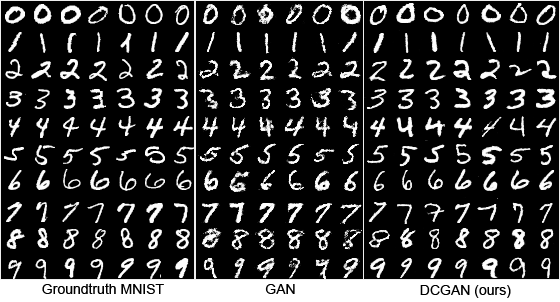## Example of GAN-generated Images @svg: media/dcgan-faces.svg 800 500 @anim: div.hasSVG | %viewbox:#zzz | %viewbox:#zzz2 # @copy:#plan: %+class:highlight: .focuses, .focus.optim ## How is all This Optimized {libyli} - Probabilistic models *{ico-pencil}* - Gibbs sampling - Expectation Maximization - Variational Inference - Black-box variational inference (e.g., [Edward](https://github.com/blei-lab/edward)) - Deep models (composition of differentiable function) - … using “back-propagation” (chain rule) - (S)GD - SGD with momentum - SGD with adaptation: RMSProp, ADAM, … - batch normalization trick - link: other tricks for [learning GANs](https://github.com/soumith/ganhacks) - are local minima any good? - link: [which optimizer?](http://sebastianruder.com/optimizing-gradient-descent/index.html#whichoptimizertouse) ## An overview of gradient descent optimization algorithms {no-print}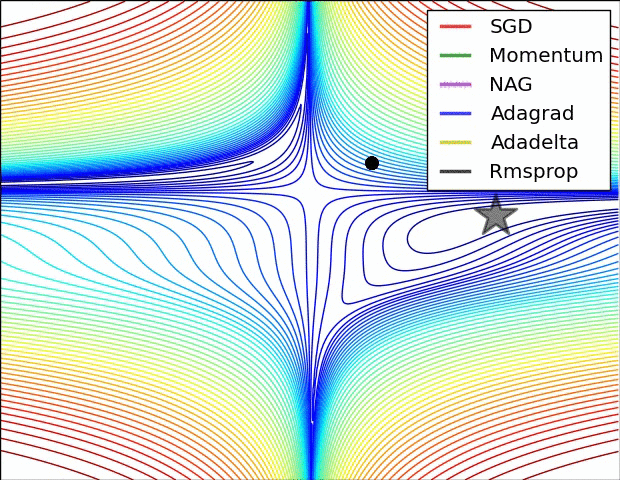[which optimizer to use?](http://sebastianruder.com/optimizing-gradient-descent/index.html#whichoptimizertouse) ## An overview of gradient descent optimization algorithms {no-print}[which optimizer to use?](http://sebastianruder.com/optimizing-gradient-descent/index.html#whichoptimizertouse) # @copy:#plan: %+class:highlight: .focuses, .focus.conv ## Convolution Models - Extensions of topic models - replace topic with motifs (with temporal structure) - PLSM, HDLSM (Emonet et al., 2014) - Convolutional Neural Networks - most of Christian's talk (ConvNets) - pixelRNN, … # @copy:#plan: %+class:highlight: .focuses, .focus.depth ## Depth in Unsupervised Learning {libyli} - Neural Network depth = Hierarchical probabilistic models - Neural Networks - “deep learning” - adding layers - handling depth with ReLU - handling depth with “ResNets”, Residual Networks (Deep residual learning for image recognition, He et al. 2015) - Hierarchical probabilistic models - Topic Models (LDA, Blei, Ng, Jordan, 2003) - Deep exponential families (Ranganath et al., 2015) *{ico-pencil}* // blei AISTATS - Deep Gaussian Processes (Damianou, Lawrence, 2013) // AISTATS # @copy:#plan: %+class:highlight: .focuses, .focus.width ## Width in Unsupervised Learning {libyli} - Width - Topic model: number of topics - Autoencoder: number of neurons in the hidden layer - GAN: size of $z$ - Non-parametric approaches, HDP, HDLSM (Emonet et al., 2014) - Gaussian process as an infinitely wide NN layer (Damianou, Lawrence, 2013) - universal function approximator - Autoencoders with group sparsity (Bascol et al., 2016) - allow for many hidden units - penalize the use of too many of them # @copy:#plan: %+class:highlight: .focuses, .focus.semantics ## Semantics in Unsupervised Learning {libyli} - Probabilistic models - inference is difficult - consider the “explains away” principle - lead to better interpretability (meaningful $z$) // rain, sun allergy, umbrella - Simpler feed-forward model - independent processing - inhibitory feedback is difficult - Bascol et al, 2016 - group-sparsity on filters - local activation inhibition - global activation entropy maximization - AdaReLU: activation function that zeroes low-energy points # @copy:#plan: %+class:highlight: .focuses, .focus.temporal ## Sequential and Temporal Modeling - cf. Christian Wolf's talk - HMM, CRF =?= RNN - LSTM =?= HSMM *{ico-hugepencil}* # @copy:#plan: %+class:highlight: .focuses, .focus.recentgans # Recent GAN Works {#recentgans} ## M5: BiGAN, ALI (2016) @svg: graphs/biganright.svg 200 500 {model} @svg: graphs/biganleft.svg 200 500 {model} *{ico-hugepencil}* ## M6: InfoGAN (2016) {libyli} @svg: graphs/infoganright.svg 250 480 {model} // @svg: graphs/ganleft.svg 93 480 {model heightauto alignbottom} - GAN noise ($z$) - is unstructured - can be partly ignored by $G$ - InfoGAN idea and principle - part of the noise is a code $c$ - enforce high mutual information
between $c$ and $\tilde{x}{}$ - in practice, predict $c$ from $\tilde{x}{}$ - use a coder $Q$ - @anim: .model - Structure in the code *{ico-pencil}* - Cartesian product of anything - (categorical, continuous, ...) - $\min_{G,Q} \max_D V_{InfoGAN}(D, G, Q) = V_{InfoGAN}(D, G) - \lambda L_I(G, Q)$ {denser} ## InfoGAN: some results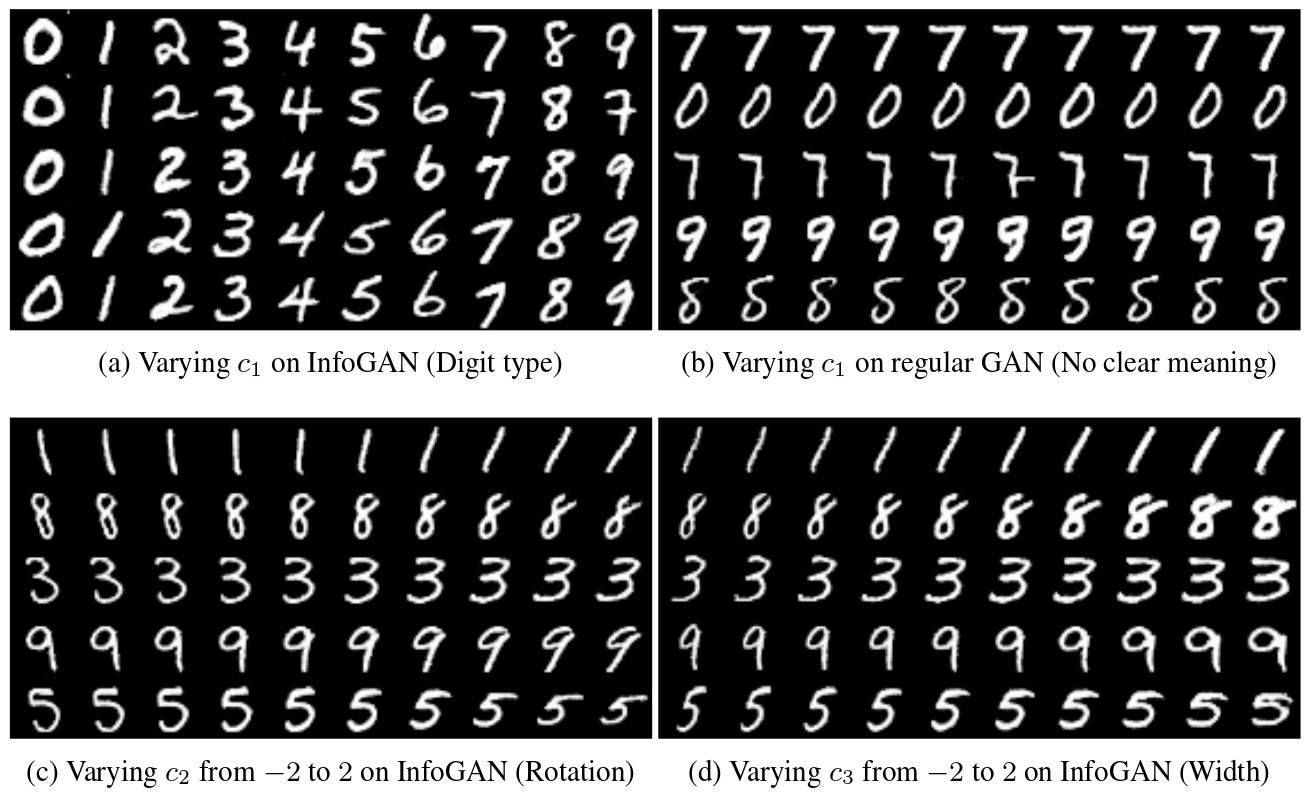## *GAN as a Modeling Tool {libyli} - Conditional GANs and variants (2016) - the GAN process is conditioned on some data - e.g., image generation condition on a semantic mask - e.g., image conditioned on a text sentence - e.g., audio conditioned on a text sentence - e.g., image conditioned on class and keypoints - … - Very complex (and operational) setups ## Ex: Learning What and Where to Draw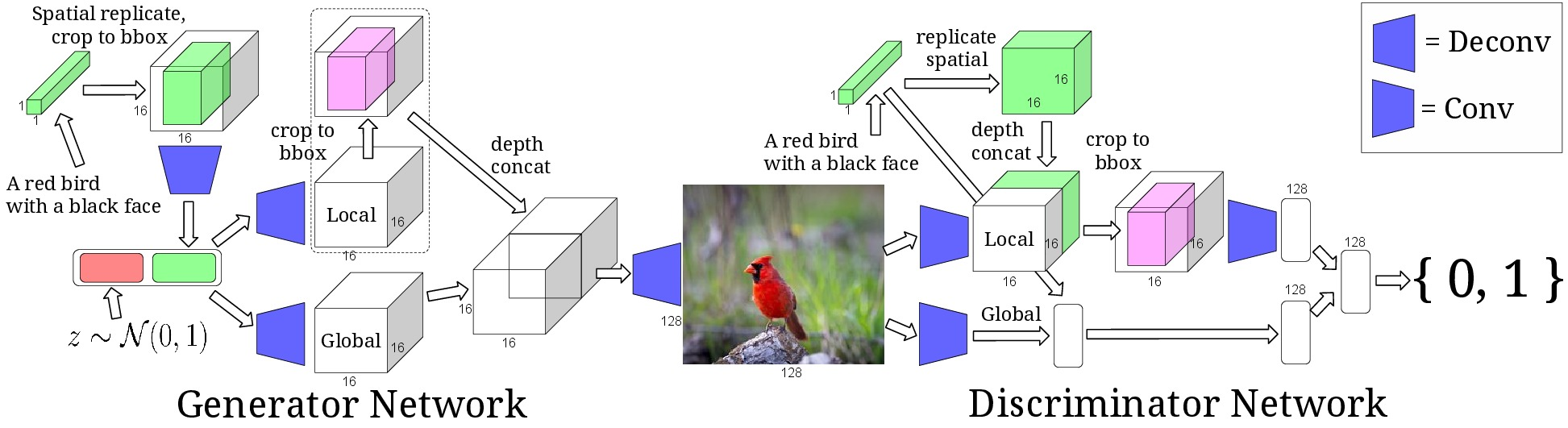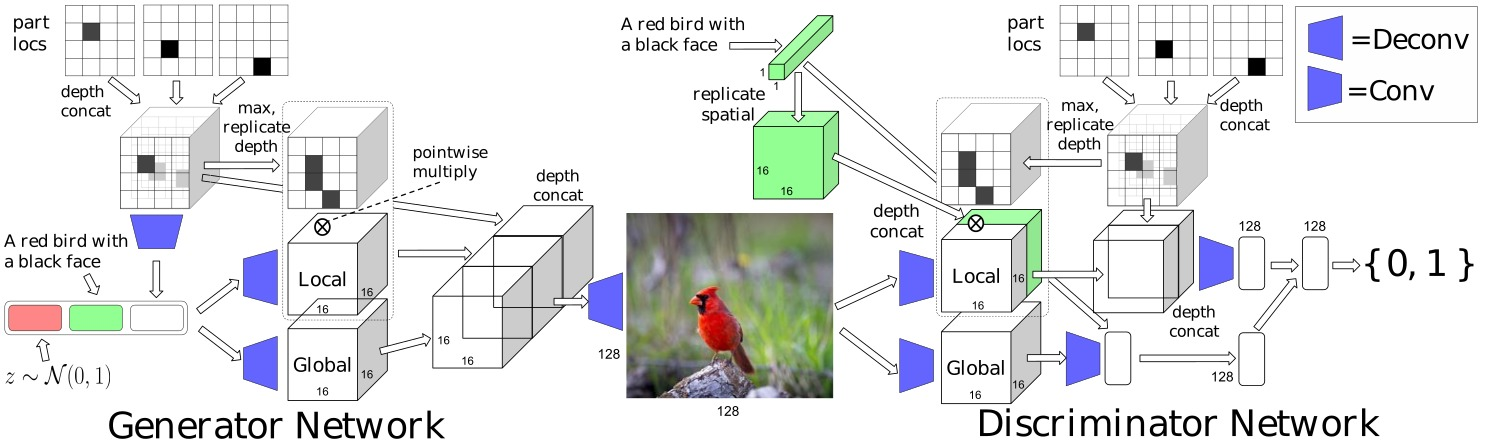## Ex: Learning What and Where to Draw - Scott Reed et al.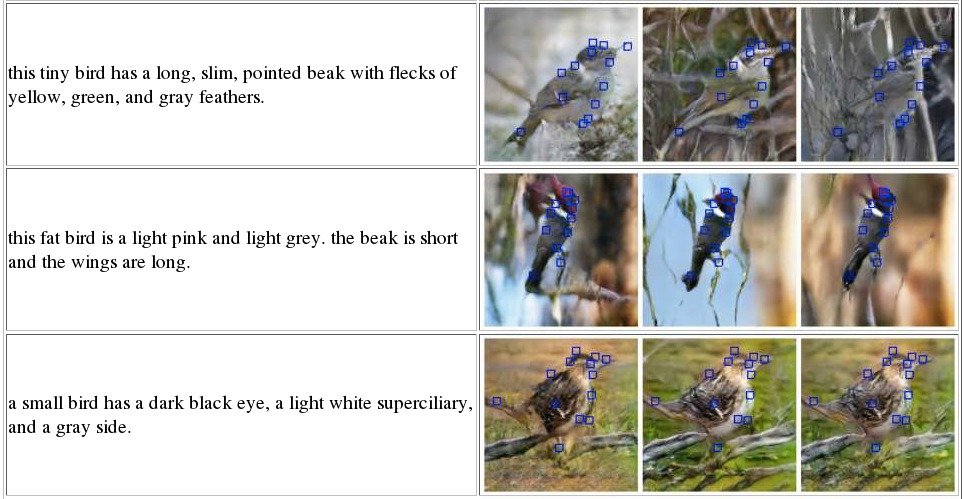# @copy:#plan: %+class:highlight: .conclusion ## Take-home Message {infobox takehome image-fit top-left darkened /black-bg /no-status /fancy-slide}
- Return of the generative approaches. - Two ways of estimating densities - generative models, - generative networks. - The golden age of Variational Inference. // (and black-box VI) - The golden age of SGD. // is the Gibbs sampling of continuous spaces - Saddle points! // active and will progress a lot ## Thank You!

Questions? {infobox image-fit top-left darkened /black-bg /fancy-slide deck-status-fake-end nocurien} - - - - twitter − [@remiemonet](https://twitter.com/remiemonet) - twitter − [@DataIntelGroup](https://twitter.com/DataIntelGroup)# Attributions ## sayumiQ {image-full bottom-left darkened /black-bg /no-status}
## seefit {image-full bottom-left darkened /black-bg /no-status}
## govan riverside {image-fit bottom-left darkened /black-bg /no-status}
## GorissenM {image-full bottom-left darkened /black-bg /no-status}
## someToast {image-fit bottom-left darkened /black-bg /no-status}
## ShutterRunner {image-full bottom-left darkened /black-bg /no-status}
## END {no-print}

/ will be replaced by the authorwill be replaced by the title